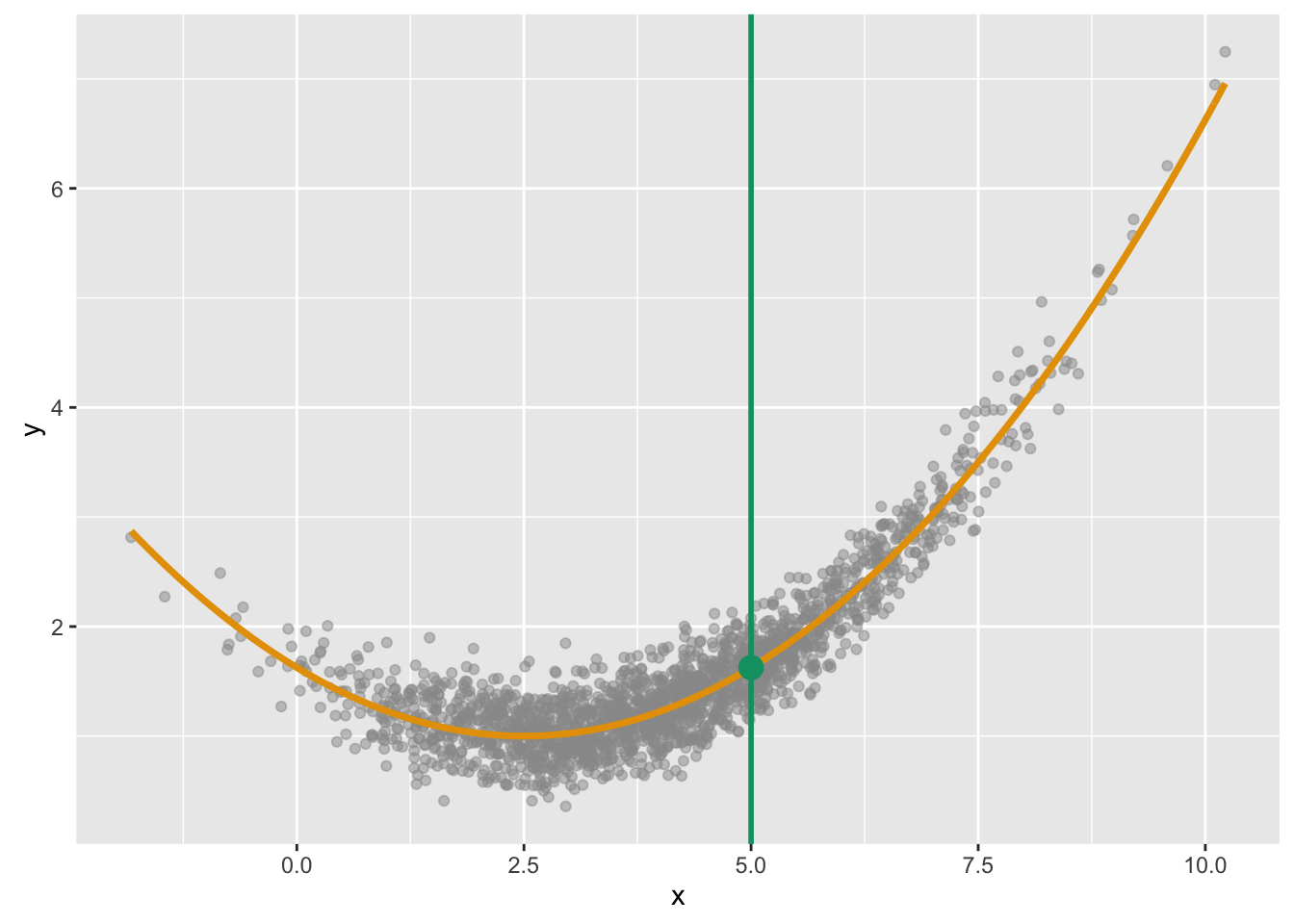### Regression function Figure

#### Data generation

# Load packages
library(ggplot2)
library(FNN)  # Used for k-nearest neighbours fit
library(ISLR)

## Note: if you get Error messages saying things like:
## Error in library(ggplot2): there is no package called 'ggplot2'
## you need to run the command:
## install.packages("ggplot2")
##
## Similarly for other packages.

# Define colour-blind-friendly colour palette
cbPalette <- c("#999999", "#E69F00", "#56B4E9", "#009E73", "#F0E442", "#0072B2", "#D55E00", "#CC79A7")

# Set seed for the random number generator
set.seed(4490)

# This is the true regression function
f.reg <- function(x) {
1 + 0.1*(x - 2.5)^2
}

# Generate data
n <- 2000 # num observations
x <- rnorm(n, mean = 4, sd = 1.75)

y <- f.reg(x) + rnorm(n, sd = 0.25)

# Combine into data frame
df <- data.frame(x = x, y = y)

x.val <- 5
y.val <- f.reg(x.val)

The code above produced 2000 randomly generated realizations from the underlying model specified by the f.reg function. More precisely, the code starts by generating 2000 x-values from the $Normal\left(4,{\sigma }^{2}={1.75}^{2}\right)$$Normal(4, \sigma^2 = 1.75^2)$ distribution, and then forms y-values according to:

${y}_{i}=1+0.1\left({x}_{i}-2.5{\right)}^{2}+{ϵ}_{i}$

where the errors are distributed as ${ϵ}_{i}\sim Normal\left(0,{\sigma }^{2}={0.25}^{2}\right)$$\epsilon_i \sim Normal(0, \sigma^2 = 0.25^2)$.

#### Resulting plots

qplot(x = x, y = y, alpha = I(0.5), colour = I(cbPalette)) +
stat_function(fun = f.reg, colour = cbPalette, lwd = 1.25) +
geom_point(aes(x = x.val, y = y.val), size = 4, colour = cbPalette) +
geom_vline(xintercept = 5, colour = cbPalette, lwd = 1)Here’s a figure showing the true regression function, the observed data, and the linear regression (blue) and 50-nearest-neighbours (green) fits to the data.

# 3-NN regression model
knn.fit <- knn.reg(train = x, y = y, k = 50)
qplot(x = x, y = y, alpha = I(0.5), colour = I(cbPalette)) +
stat_function(fun = f.reg, colour = cbPalette, lwd = 1.5) +
stat_smooth(method = "lm", se = FALSE, lwd = 1) +
geom_line(aes(x = x, y = knn.fit\$pred), colour = I(cbPalette), lwd = 0.75)